Courses

# JEE(MAIN) Mathematics Mock Test - 7

## 30 Questions MCQ Test JEE Main Mock Test Series 2020 & Previous Year Papers | JEE(MAIN) Mathematics Mock Test - 7

Description
This mock test of JEE(MAIN) Mathematics Mock Test - 7 for JEE helps you for every JEE entrance exam. This contains 30 Multiple Choice Questions for JEE JEE(MAIN) Mathematics Mock Test - 7 (mcq) to study with solutions a complete question bank. The solved questions answers in this JEE(MAIN) Mathematics Mock Test - 7 quiz give you a good mix of easy questions and tough questions. JEE students definitely take this JEE(MAIN) Mathematics Mock Test - 7 exercise for a better result in the exam. You can find other JEE(MAIN) Mathematics Mock Test - 7 extra questions, long questions & short questions for JEE on EduRev as well by searching above.
QUESTION: 1

Solution:
QUESTION: 2

Solution:
QUESTION: 3

### The area contained between the curve x y = a2 , the vertical line x = a, x = 4a (a > 0) and x -axis is

Solution:
QUESTION: 4

A circle passes through (0,0) and its centre lies on y=x. If it cuts the circle x2+y2-4x-6y+10=0 orthogonally, then its equation is

Solution:
QUESTION: 5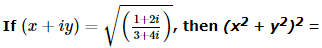Solution:
QUESTION: 6

In the following question, a Statement of Assertion (A) is given followed by a corresponding Reason (R) just below it. Read the Statements carefully and mark the correct answer-
Let n ≥ 3 and let the complex numbers α1 , α2 , . . . , αn be the roots of xn − 1 = 0 with α1 = 1 .
Assertion(A) :For any positive integer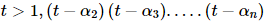is again a positive integer.
Reason(R) :For any positive integer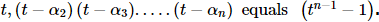Solution:
QUESTION: 7
The solution of the equation |z| - z = 1 + 2i is
Solution:
QUESTION: 8
For solving dy/dx=4x+y+1 suitable substitution is
Solution:
QUESTION: 9

If y=x2(x-2)2, then the values of x for which y is increasing, are

Solution:
QUESTION: 10

When y = 3, which of the following is FALSE?

Solution:

Remember what is TRUE: 3 is prime, odd and 2(3) is even.
Choice 1: T and T is TRUE
Choice 2: T or F is TRUE
Choice 3: F and T is FALSE
Choice 4: T and T is TRUE

QUESTION: 11

Let the function f be defined by f(x) = 2x + 1/1- 3x. Then f⁻1(x) is

Solution:
QUESTION: 12

In the following question, a Statement of Assertion (A) is given followed by a corresponding Reason (R) just below it. Read the Statements carefully and mark the correct answer-
Assertion(A): If f : R → R defined by f (x) = x3 then f is one one onto
Reason(R) : Function f is strictly decreasing on R.

Solution:
QUESTION: 13

In the following question, a Statement of Assertion (A) is given followed by a corresponding Reason (R) just below it. Read the Statements carefully and mark the correct answer-
Assertion(A): y = sin (ax + b) is a general solution of y" + a2 y = 0 .
Reason(R) : y = sin (ax + b) is a trigonometric function.

Solution:
QUESTION: 14

If A is a square matrix such that A2 = I, then A⁻1 is equal to

Solution:
QUESTION: 15

If x+y+1=0 tocuhes the parabola y2=λx,then λ is equal to

Solution:
QUESTION: 16

If P(B)=(3/4), P(A∩B∩C̅) = (1/3) and P(A̅∩B∩C̅) = 1/3, then P(B∩C) is

Solution:
QUESTION: 17

A polygon has 44 diagonals. The number of its sides is

Solution:
QUESTION: 18

Given n = 10, ∑x = 4, ∑y = 3, ∑x2 = 8, ∑y2 = 9 and ∑xy = 3, then coefficient of correlation is

Solution:
QUESTION: 19
The equation of sides of a quadrilateral AB, BC, CD and DA are x+2y=3, x=1, x-3y=4, 5x+y+12=0, the angle between diagonals AC and AD is
Solution:
QUESTION: 20

The straight line x + y = a will be a tangent to the ellipse x2/9 + y2/16 = 1 if a =

Solution:
QUESTION: 21

The shortest distance from the point (1, 2, -1) to the surface of the sphere x2 + y2 + z2 = 24 is

Solution:
QUESTION: 22

If cos (A - B)=3/5 tan A tan B=2, then

Solution:
QUESTION: 23

The angle between the vectors 3i+j+2k and 2i-2j+4k is

Solution:
QUESTION: 24

If the dot product of vector i+j+k and the unit vector along the sum of vectors 2i+4j-5k and λi+2j+3k is 1, then λ=

Solution: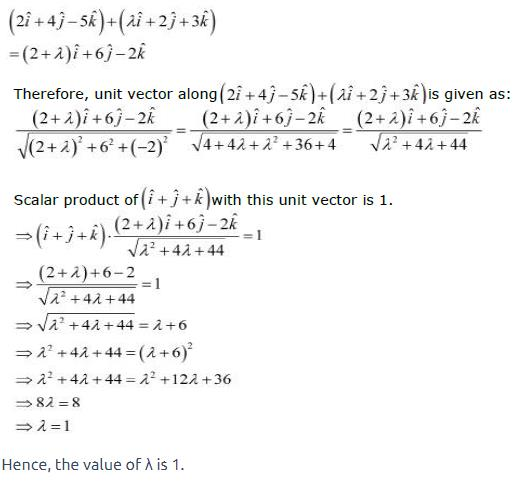QUESTION: 25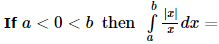Solution:
QUESTION: 26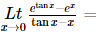Solution:
QUESTION: 27

In the following question, a Statement of Assertion (A) is given followed by a corresponding Reason (R) just below it. Read the Statements carefully and mark the correct answer-
Assertion(A):If A and B are two independent events such that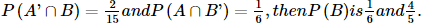Reason(R):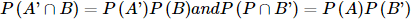Solution:
QUESTION: 28

If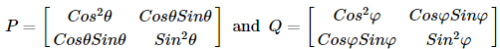are two matrices such that the prouduct PQ is null matrix, then θ − φ is

Solution:
QUESTION: 29

Consider an infinite geometric series with first term a and common ratio r. if its sum is 4 and the second term is 3/4 ,then

Solution:
QUESTION: 30

The largest value of 2x3 - 3x2 - 12x + 5 for - 2 ≤ x ≤ 4 occurs at x =

Solution: